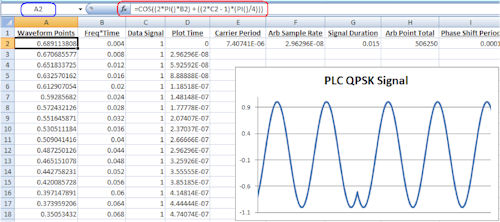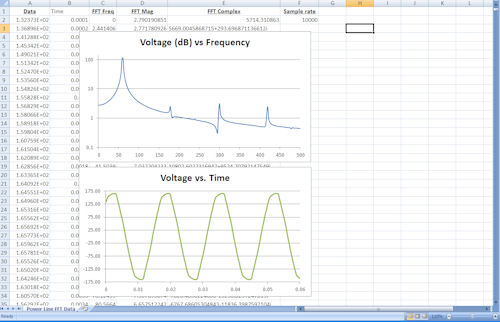# Striving for Excel-lence

## How to use Excel to avoid writing code for test instruments.

How to use Excel to avoid writing code for test instruments.

#### By Neil Forcier, Agilent Technologies

When you are working on your bench and you need to create an arbitrary waveform, or you need to post-process measurement data, it usually means you need to write some code or buy a software package. Both these approaches can be costly and time-consuming, however, and can cause your design momentum to evaporate abruptly.

In the benchtop test environment, you often can avoid the interruption by simply combining the capabilities of Microsoft Excel with the easy data transfer capabilities found in modern test-and-measurement instruments,  and free test-and-measurement software packages that are readily available. Let’s look at some common bench test situations where Excel makes an easy-to-use substitute for writing a custom program or using purchased software. Most engineers who use Excel view it as a tool for tracking parts, managing design budgets or creating plots of data for test reports. In all these cases, just the general functionality of Excel is used: organizing data in rows and columns, basic arithmetic operations and plotting. But Excel also offers built-in advanced mathematical tools, such as sine and random number functions, as well as the ability to do Fast Fourier transforms (FFTs).

Creating Waveforms and Signals in Excel

Let’s start with a common situation: creating an arbitrary waveform and getting it onto an arbitrary waveform generator. For our example, let’s say we need to create a quadrature phase-shift-keyed (QPSK) signal for doing a power line communication (PLC) test. For our example PLC signal, we will use a carrier frequency of 135 KHz. The bit rate of the data is 20 Kb/s, and we want the signal to run for 15 ms. If we choose to have 250 points in each carrier frequency cycle, our waveform will consist of 506,250 points. See the equation for a QPSK signal in Figure 1.Figure 1: QPSK signal equation.
Figure 2 shows the QPSK signal waveform created using Excel. The data points for the finished waveform that we will upload are in column A. The equation used to calculate each data point at A2 is =COS((2*PI()*B2+((2*C2-1)*(PI()/4))), which was entered into Excel’s data entry box.Figure 2: QPSK signal in Excel.
Excel has a library of built-in functions, like cosine and standard deviation. To apply a function or any mathematical operation to a highlighted cell, simply start with an “=” in the data entry box. If you do not know the Excel “command” for the built-in function you want to use, simply press the “fx” button next to the data entry box to search for a specific function.If you examine the function of the QPSK signal that was typed into Excel’s data entry box” =COS((2*PI()*B2+((2*C2-1)*(PI()/4)))”  besides the cosine function, numbers, and mathematical operators, you will notice some non-number variables like “PI(),” “B2” and “C2.” PI() is simply a built-in function that represents the number pi. B2 and C2 are variables representing the number values in those corresponding cells on the spreadsheet. Column B contains the multiple to create a full cycle every 250 points. In column C is the data signal that can take on four different values, 1 through 4; each value represents two bits of data.To create the timing data in column B, we used a simple formula to increment the value in the proceeding cell by 0.004. To build the baseband data in column C, we used Excel’s built-in RANDBETWEEN() function. It allowed us to randomly generate numbers between 1 and 4.Copy and PasteNow that you understand how the calculations were made,  let’s look at how to make more than 500,000 copies of the calculations and data. To move the function that was created for cell A2, to the other cells in the A column, we can simply copy and paste. Excel is smart enough to know that if you use B2 and C2 in a function for A2, and you paste that same function into A126, it will increment the variables to B126 and C126.Because we are working with a large amount of data, it is not possible to individually paste to each cell or even highlight drag and paste. Excel provides multiple ways to handle large amounts of data. One way to jump to different cells is to type the cell you want selected into the cell box to the left of the data entry box near the top of the Excel window. To select or highlight a large number of cells to paste a function, say, 506,249 times,  simply start at A3, press “Select,” and then type A506251 in the cell box. At this point, we are ready to move the QPSK signal waveform to an arbitrary waveform generator. To do this, first save the Excel file (file type .xlsx) to a comma-separated value (CSV) file (file type .csv), which is the file type modern arbs can read. For this example, we used an Agilent 33521A function/arbitrary waveform generator. We put the QPSK CSV file on a memory stick, which we plugged into the 33521A’s front-panel USB slot. The QPSK signal waveform was then uploaded and outputted by the 33521A.A scope screen capture of a portion of the QPSK signal waveform can be seen in Figure 3 on page 28.Figure 3: QPSK signal created in Excel.

Post-processing Data

Let’s look at an example of the reverse case, where we want to pull data off a measurement instrument and post-process it in some way for further analysis. For this example, we want to digitize a 60-Hz power line signal and then perform an FFT to analyze its harmonic distortion.We used an Agilent 34411A digital multimeter (DMM) for this measurement. The 34411A has a built-in low-frequency digitizer capability “and because it is a DMM, it has high resolution for detailed insight into the measured signal. We made 4,096 measurements on the power line signal at a sample rate of 10 KS/s. To get from the DMM to Excel without any code, we used the 34411A’s LXI Web interface. The built-in Web interface allows you to control and fetch readings from the instrument via a Web browser. To transfer the readings from the text field of the Web interface, we copied them and pasted them into Notepad. We then saved the Notepad file as a CSV file and opened it in Excel.To do the FFT, we used Excel’s data analysis tool set, which guides you through performing an FFT on a data set “and also includes various other engineering and statistical tools. For step-by-step instruction on performing an FFT in Excel, simply search the Web for “Excel FFT” and plenty of tutorials will show up. Figure 4 shows the captured power line signal data,  with both a frequency-domain plot (using the FFT data) and a time-domain plot.Figure 4: Screen shot of Excel FFT.

Create Test Scripts on the Cheap

Free software is available for creating test scripts or sequences in Excel that allow you to connect and control instruments without writing code. For this example, we used a free software package called Command Expert. When you download Command Expert, it creates a plug-in to Excel that allows you to easily build instrument control scripts with, of course, no programming required. Figure 5 shows a screen shot from a script created with Command Expert that connects to a signal analyzer, makes a series of frequency and power measurements based on a user-defined frequency range, and displays the resulting data.Figure 5: Excel test script created with Command Expert.
Combing software packages like Command Expert with Excel is a great way to create simple automated tests with no programming and no added cost.

Putting it All Together

In this article, we looked at how you can combine Excel with modern instrument features and free software packages to provide an alternative to time-consuming programming and costly software. We looked at Excel’s built-in advanced mathematical and data analysis tools for processing or post-processing data that we want to push onto an instrument (in the case of the QPSK waveform), or pull from an instrument (in the case of the signal data). Modern instrument features, such as Web interfaces and USB drives, allow us to easily move data between Excel and the instrument. Finally, we looked at how free software packages, like Command Expert, can be used with Excel to create simple automated tests without writing code. While it’s not ideal for every situation, there are a number of applications in the benchtop test environment where using Excel can avoid delays.

Neil Forcier served in the U.S. Navy as an electronic test equipment calibration technician on board the USS Harry S Truman CVN-75. He earned his bachelor’s degree in engineering from the Pennsylvania State University, University Park campus. He is currently working as an application engineer for the System Products Division at Agilent Technologies. Send e-mail about this article to [email protected].

Agilent Microsoft

## Subscribe to our FREE magazine, FREE email newsletters or both!

Join over 90,000 engineering professionals who get fresh engineering news as soon as it is published.DE Editors

DE’s editors contribute news and new product announcements to Digital Engineering. Press releases can be sent to them via [email protected].

## Related Topics

Test   Agilent Technologies   Instrumentation   All topics
#2808